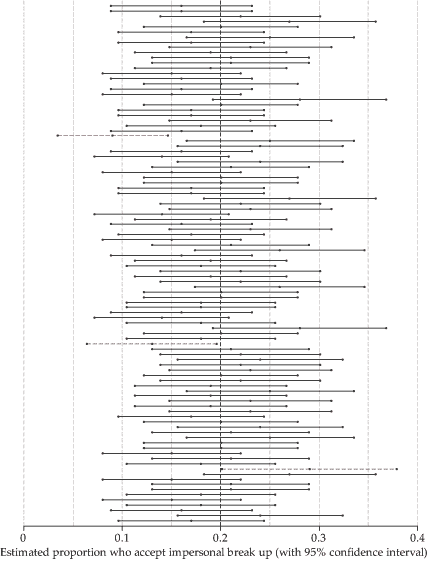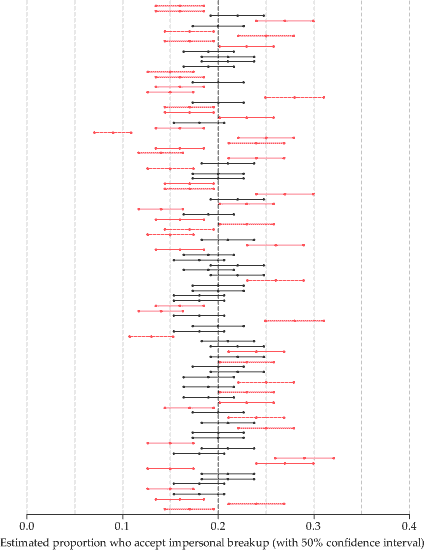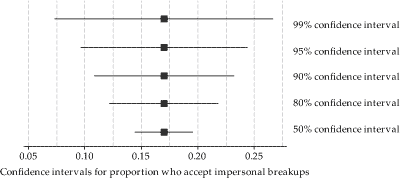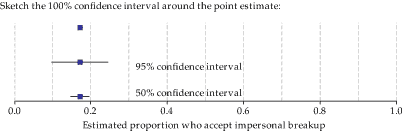## Content

### Confidence intervals

This section deals with fundamental aspects of confidence intervals. In the next section, we will deal with obtaining a confidence interval for the specific case we are considering. But it is important first to understand confidence intervals conceptually.

An observed sample proportion $$\hat{p}$$ is a single point or value that provides us with an estimate of the true proportion of interest in the population. For this reason, it is called a point estimate. In some sense, we are not interested in the particular value of the sample proportion per se, but rather we are interested in the information it provides us about the population. It provides an estimate of the population parameter of interest; in this case, the population proportion $$p$$.

While the proportion from the sample will provide us with the best estimate of the population proportion, it is unlikely that the sample value will be exactly equal to the parameter being estimated. Hence, the sample estimate is most useful if it is combined with some information about its precision.

Suppose, for example, we want to estimate $$p$$, the proportion of Australian adults aged 18–24 who feel that it is acceptable for a breakup to be conveyed via email, phone or text message (for brevity, we refer to this as an 'impersonal' breakup), and that we have the results of two different surveys on hand, each of them based on random samples. The first survey provides an estimate of the proportion equal to 0.17 (17%), while the second survey provides the estimate 0.28 (28%). These estimates may seem inconsistent, and it may be unclear which we might prefer to rely on. However, if the first survey result is likely to be within $$\pm 0.07$$ of the true value of $$p$$, and the second survey result is likely to be within $$\pm 0.25$$ of the true value of $$p$$, then the first result is more precise than the second.

By describing the first survey result as $$0.17 \pm 0.07$$, we are specifying an interval or range of values (from $$0.17-0.07$$ to $$0.17+0.07$$) within which we have confidence that the true value of $$p$$ lies. The interval has a lower bound and an upper bound: 0.10 and 0.24, respectively. This interval is an indicator of the precision of an estimate of the population proportion and is called a confidence interval. Here the confidence interval is $$(0.10, 0.24)$$.

Although we are discussing the specific context of an inference on $$p$$, most of the ideas discussed in this section apply to confidence intervals for any unknown population parameter.

'Confidence' has a particular meaning in this context, which we now describe.

#### Confidence level

When working out a confidence interval, we must first decide on a 'degree' or 'level' of confidence. This is quantified by the confidence level. We want to be very confident, so it makes sense to have a high confidence level. In most applications, the confidence level used is 95%. This is a very strong tradition. You may wonder: Why don't we use 100%, and work out a 100% confidence interval? This question is asked in exercise 3.

A confidence interval will always be obtained from a random variable, so the interval itself can be thought of as a random interval. It varies from one sample to the next, just as a random variable does.

The confidence level specifies the long-run percentage or proportion of confidence intervals containing the true value of the parameter: in this context, $$p$$. Illustrating this idea requires a simulation or a thought experiment. In practice, we typically do not have a long run of repeated samples at all. We have a single sample of size $$n$$, and we calculate $$\hat{p}$$ and a single confidence interval to characterise the precision in the result. Any actual interval either contains or does not contain the true value of the parameter $$p$$, although we don't know whether it does or not, because we don't know the value of $$p$$. For example, we don't know whether the interval 0.10 to 0.24, for the proportion of Australian adults aged 18–24 who feel that impersonal breakups are acceptable, contains the true value $$p$$. The confidence level of 95% being used here does not mean that the chance of this particular interval containing $$p$$ is 95%.

To illustrate the meaning of the confidence level, think about the true proportion $$p$$ of Australian adults aged 18–24 who feel that impersonal breakups are acceptable. As we observed, we don't know the value of $$p$$; estimating $$p$$ is what we are trying to do! Assume, just for the purposes of this discussion, that $$p=0.20$$ (20%). The first survey described above is based on a random sample of 100 adults aged 18–24, and 17% indicated that an impersonal breakup is acceptable. We can imagine repeating the process used in the first survey many times, sampling different adults each time, while maintaining random sampling and a sample size of $$n=100$$. Each time we will observe a different sample proportion.

figure 11 shows 100 such surveys, with the first survey result closest to the horizontal axis. For each survey, the estimate of the proportion of interest is plotted as a dot in the centre of a line. The line shows the 95% confidence interval for the particular survey. For the first survey, the line showing the 95% confidence interval is from 0.10 to 0.24.Detailed description

Figure 11: One hundred surveys, based on random samples of 100 adults aged 18–24, estimating the proportion who regard impersonal breakups as acceptable, showing the point estimate and the 95% confidence interval in each case.

figure 11 shows a darker vertical gridline, corresponding to the true value of $$p$$, namely $$p=0.20$$. Most of the confidence intervals are colored black, but a small number are red; these are the confidence intervals that do not include the true value of 0.2. In total, three of the one hundred intervals are red. There are two on the low side, where all values in the interval are less than 0.2, and one on the high side, where the lower bound of the interval is greater than 0.2. In this small simulation, 97% of the intervals include the true value. We expect that 95% of the 95% confidence intervals will include the true value; with much larger simulations, the percentage would be very close to 95%.

Thinking about this more formally, if we define $$\mathrm{CI}_{0.95}(\hat{P})$$ to be the 95% confidence interval that is based on the random variable $$\hat{P}$$, then we can write

$\Pr[p \in \mathrm{CI}_{0.95}(\hat{P})] = 0.95.$

We have expressed this in the specific context we are considering, but the point is general: A 95% confidence interval (considered as a random interval) for an unknown parameter has a probability of 0.95 of containing the parameter.

##### Exercise 2

Suppose we have $$m$$ independent 95% confidence intervals for an unknown parameter.

1. Define $$Y$$ to be the number of intervals that include the unknown parameter value. What is the distribution of $$Y$$? (Hint. Think of the $$m$$ intervals as a sequence of Bernoulli trials.)
2. Hence, what is $$\mathrm{E}(Y)$$?
3. Now assume $$m=100$$.
1. Find the chance that exactly 95 of the intervals include the parameter, that is, find $$\Pr(Y = 95)$$.
2. Find the chance that at least 95 of the intervals include the parameter.

#### Varying the confidence level

figure 12 represents the same 100 surveys of 100 Australian adults aged 18–24. For each survey, the estimate of the proportion of interest is plotted as a dot in the centre of a line which this time shows the 50% confidence interval. The central dot is the point estimate. Hence, because they are the same surveys, the central dots in figure 12 are at the same positions as those in figure 11.

However, the confidence intervals are much narrower. As before, the confidence intervals shown in black contain the true value of the parameter, namely $$p=0.2$$, and those shown in red do not. The 50% confidence intervals are narrow and therefore may appear precise. But figure 12 indicates that this is at a price. About half of them do not include the true value of interest.Detailed description

Figure 12: One hundred surveys, based on random samples of 100 adults aged 18–24, estimating the proportion who regard impersonal breakups as acceptable, showing the point estimate and the 50% confidence interval in each case.

Another way to see the effect of varying the confidence level is to examine confidence intervals with different confidence levels for the same survey. This is shown in figure 13, using the first survey of 100 Australian adults aged 18–24. The confidence intervals have different confidence levels. Since the same survey is represented in each case, the point estimate is the same, but the confidence intervals have different widths.Figure 13: Confidence intervals from the same data, but with different confidence levels.

##### Exercise 3

Consider the following figure, which shows the 50% and 95% confidence intervals for the proportion of Australian adults aged 18–24 who feel that impersonal breakups are acceptable, based on the first survey. Sketch the 100% confidence interval on the figure.Detailed description

Figure 14: Estimate of the proportion who regard impersonal breakups as acceptable from one survey.

We have discussed the general properties of a confidence interval. We now turn to the practical issue of obtaining a confidence interval for the unknown population proportion $$p$$.

Next page - Content - Calculating confidence intervals